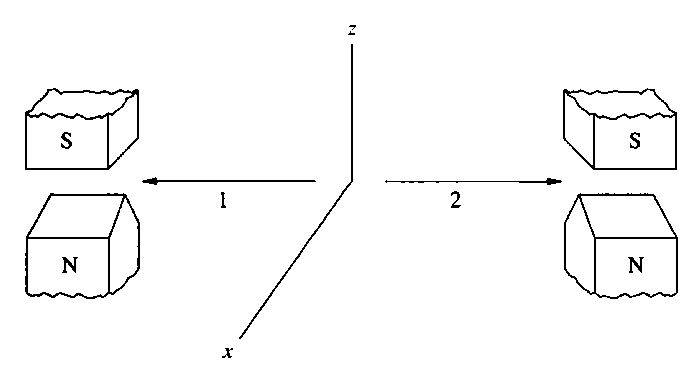# Quantum entanglement of spin along multiple orthogonal axes

I already asked this question on physics.stackexchange.com, but did not get the desired response. I am interested in the opinion of your community.
Picture an entangled pair of spin 1/2-spin particles with total spin 0. In the diagram, particle 1 of the pair is moving to the left (-y), and particle 2 to the right (+y).

If a z-oriented SG is used to detect the spin direction of particle 1 on the left, then the spin direction of particle 2 can be predicted with 100% certainty by using another z-oriented SG on the right. For example, if the left SG finds particle 1 to have spin $\frac{\hbar}{2}$, there is be a 100% probability that a z-directed SG on the right will detect particle 2 as having spin $-\frac{\hbar}{2}$.

Now consider leaving the left SG unchanged (pointing to +z), and rotating the right SG so it will point to +x. If particle 1 is detected on the left to have spin $\frac{\hbar}{2}$, two possibilities can be considered for what will happen when particle 2 reaches the +x-directed SG:

- Particle 2 is detected with 100% probability as having spin $-\frac{\hbar}{2}$ in the +x-direction, or

- Particle 2 is detected with a 50% probability of having spin $-\frac{\hbar}{2}$ in the +x direction, and 50% of having spin $+\frac{\hbar}{2}$ in the -x direction.

In the second case, obviously, it may turn out that the total spin of the system is not equal to zero.

What is the result of measurement, we should expect from SG{+x}?

*SG: Stern-Gerlach apparatus## Answers and Replies

Bill_K
Science Advisor
Since you already have a "desired response" I wonder why you are bothering to phrase it in the form of a question. After all of the responses are received, please let us know what grade we got.

Particle 2 is guaranteed to be in the state sz = -ħ/2. Particle 1 is now out of the picture. As always, when you measure sx on this state you'll get +ħ/2 and -ħ/2 each with 50% probability.

Particle 2 is guaranteed to be in the state sz = -ħ/2. Particle 1 is now out of the picture. As always, when you measure sx on this state you'll get +ħ/2 and -ħ/2 each with 50% probability.

I thank you for your answer. Let we assume, we will find first one particle in +z state, and second one particle , for example, in +x state with $+\frac{\hbar}{2}$ spin. Does this mean the total spin of entangled pair after measurement became unequal to zero?
If this is true, Is there not broken the law of angular momentum concervation?

Bill_K
Science Advisor
Interaction with the Stern-Gerlach apparatus causes the angular momentum of the particle to be changed. If you take a particle which is initially sz = +ħ/2, do a sx measurement and observe it to be sx = +ħ/2 say, then follow it with another sz measurement, the particle can become sz = -ħ/2.

Interaction with the Stern-Gerlach apparatus causes the angular momentum of the particle to be changed. If you take a particle which is initially sz = +ħ/2, do a sx measurement and observe it to be sx = +ħ/2 say, then follow it with another sz measurement, the particle can become sz = -ħ/2.

Ok, Bill_K, thanks a lot. The quastion became clear for me.